# Why does light bend on refraction?

• ajay.05

#### ajay.05

This may be silly...but why does light bend on moving from one medium to another medium. i can understand that, the density of particles, make it difficult for light to travel, hence it slows down. But why should it bend?

hence it slows down. But why should it bend?
The slowing causes the bend:

http://en.wikipedia.org/wiki/Huygens–Fresnel_principle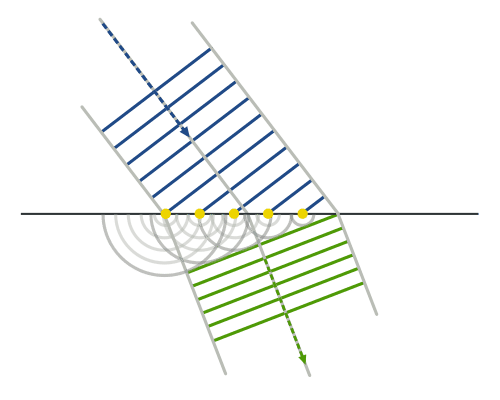The slowing causes the bend:

http://en.wikipedia.org/wiki/Huygens–Fresnel_principle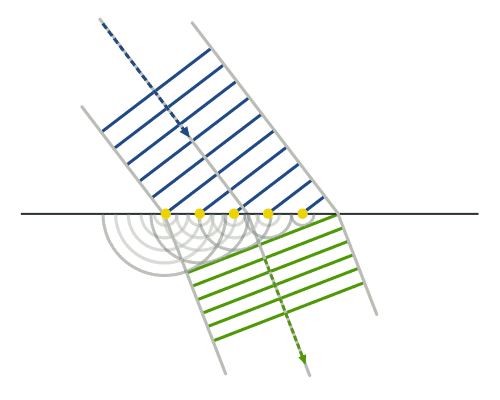Can you please elaborateCan you please elaborateCan you please elaborateThe Huygens-Fresnel principle is so important to these problems that, no matter how much we eleborate, you'll want to study it for yourself. If you find the wikipedia article a bit heavy going, google will find you some more easily understood explanations and examples.

•Doug Huffman
Can you please elaborateI think in the Huygens picture, the requirement is completely geometrical. The semi circles are the wavelets that are emitted at every point on the wavefront. On the incident surface, points on the incident wavefront arrive at different times and the wavelets are thus considered to emerge at different instants.

Under such construction, you could construct triangles from the distance traveled by the left wavelet and the incident surface. From that you could determine the direction of travel.

Incidentally, for refraction of light, you could also solve simple Maxwell's equations in dielectric medium. By matching the boundary conditions, you could get the relationships between incident and refracted E-M fields. Wave vector could be related using Maxwell's equation. But this is not recommended for people unfamiliar with E-M.

I was in the same situation some time back, trying to understand reason for refraction of wave.
I will share with you my findings.

First thing, I don't think the video above gives the right explanation.
Since if you try to find out the reason for the observed behavior in the above video
you will see that one wheel wants to go with faster speed and other slower.
Due to which a force on faster wheel acts towards the slower wheel and eventually as the result of all the mechanics happening, the direction of wheel gets changed.
but none of such thing happens in case of wave. No wave exerts a force on another,
or alters the motion of any other wave in any way.
Also if we perform the above experiment with cycle, then we will see that it doesn't bend.

So what does really happens then?

Basically the first important point here to understand is that a wave is in constant urge of bending or
This is clearer if we perform a simple experiment, where we let the wave pass through a hole.
We see that as the wave passes the hole, it spreads uniformly as shown in diagram below.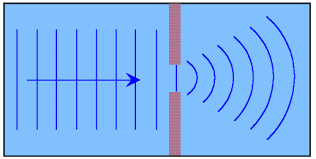This is also known as diffraction.

This same urge of spreading is there all the times (known as Hygen's principle), but is not observed earlier (before reaching the hole)
as the urge to spread by wave at a point is canceled by the urge to spread by the wave at the adjacent point
and thus ultimately as a result, the whole wave just moves forward.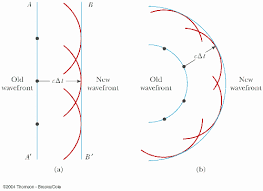Now, if we do two holes,
then we can notice that wave reaches one hole earlier and the other hole later.
The wave after reaching the first hole spreads in all direction until the wave from the second hole arrives and
the combined effect comes into play. So this in all means that during the time second wave arrives,
first wave get the chance to spread freely and this leads to refraction, where you consider there are infinite adjacent such holes.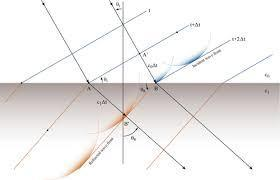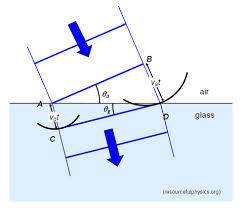Hope this helps.

•ajay.05
I was in the same situation some time back, trying to understand reason for refraction of wave.
I will share with you my findings.

First thing, I don't think the video above gives the right explanation.
Since if you try to find out the reason for the observed behavior in the above video
you will see that one wheel wants to go with faster speed and other slower.
Due to which a force on faster wheel acts towards the slower wheel and eventually as the result of all the mechanics happening, the direction of wheel gets changed.
but none of such thing happens in case of wave. No wave exerts a force on another,
or alters the motion of any other wave in any way.
Also if we perform the above experiment with cycle, then we will see that it doesn't bend.

So what does really happens then?

Basically the first important point here to understand is that a wave is in constant urge of bending or
This is clearer if we perform a simple experiment, where we let the wave pass through a hole.
We see that as the wave passes the hole, it spreads uniformly as shown in diagram below.
View attachment 77041
This is also known as diffraction.

This same urge of spreading is there all the times (known as Hygen's principle), but is not observed earlier (before reaching the hole)
as the urge to spread by wave at a point is canceled by the urge to spread by the wave at the adjacent point
and thus ultimately as a result, the whole wave just moves forward.Now, if we do two holes,
then we can notice that wave reaches one hole earlier and the other hole later.
The wave after reaching the first hole spreads in all direction until the wave from the second hole arrives and
the combined effect comes into play. So this in all means that during the time second wave arrives,
first wave get the chance to spread freely and this leads to refraction, where you consider there are infinite adjacent such holes.

View attachment 77042View attachment 77043

Hope this helps.
Thank you! Well Explained!
I'm out of my doubts now.

Last edited:
An alternative explanation would be Fermat's Principle of Least Time. It states that, two go between two given points light will take the path which takes the least time. You can set up a situation where light has to start at one side of a glass block and has to go to a point in the other side offset from the first point by some distance. Using the principle of least time you can work out that , because light slows down in the block, to get to its destination in the least possible time, the light will have to bend.

I don't find this explanation correct. It doesn't explain reflection. Please correct me if i am wrong.

Actually it does. Say you have a source of light near the mirror, some of the light rays will go from a to b in a straight line. But some rays, because of the direction in which their emitted will hit the mirror. So if you put the condition that the light has to hit the mirror, you'll be able to derive the law for reflection using the principle of least time.

Say you're standing in front of a mirror with a lantern in your hand( god knows why). The light from the lantern reaches your eyes in two ways: straight from the lantern and after having reflected of the mirror.
So if you constrain light to hit the mirror, then the path it will to reach a certain point in the least time will be such that the angle if incidence equals the angle of reflection.